GeeksforGeeks App
Open AppBrowser
Continue

# Palindromic Tree | Introduction & Implementation

Palindromic Tree is a tree-based data structure designed for efficient string processing tasks such as pattern matching, string searching, and spell-checking. It is an advanced data structure based on a trie that is optimized for palindromic strings.

A Palindromic Tree is a trie-like data structure that stores all the substrings of a given string in such a way that it can efficiently handle string queries such as finding the longest palindromic substring or counting the number of palindromic substrings. It works by maintaining a tree of all the palindromic substrings of the input string.

A palindromic tree is a data structure used in string algorithms and computer science. It’s designed to efficiently process strings and identify palindromes within them.

### Introduction:

Definition: A palindromic tree is a type of Trie data structure that stores strings and efficiently identifies all the palindromic substrings within them.
Purpose: The palindromic tree is used in a variety of applications, including text processing, string matching, and pattern recognition.
Characteristics: A palindromic tree has two key characteristics – it’s a tree structure, and it’s optimized for efficiently processing palindromic substrings.

### Node Structure:

Definition: Each node in a palindromic tree represents a substring of the input string.
Components: Each node has several components, including a pointer to its parent node, pointers to its children nodes, and a length attribute representing the length of the substring represented by the node.
Suffix Link: Each node in a palindromic tree also has a “suffix link,” which is a pointer to another node that represents a suffix of the substring represented by the current node.

### Palindrome Identification:

Definition: A palindrome is a string that reads the same forwards and backwards.
Identification: The palindromic tree uses a series of algorithms and rules to identify all the palindromic substrings within a given input string.
Rule-Based Approach: One of the key approaches used by the palindromic tree to identify palindromes is a rule-based approach, which uses a set of rules and algorithms to determine whether a given substring is a palindrome.

### Insertion:

Definition: The insertion process in a palindromic tree involves adding new nodes to the tree structure to represent new substrings in the input string.
Algorithm: The insertion process in a palindromic tree is performed using a series of algorithms that build new nodes and add them to the tree structure as needed.
Extension: The process of inserting a new node into a palindromic tree involves extending the tree structure to accommodate the new substring, and updating the tree’s links and relationships as necessary.

### Query Processing:

Definition: Query processing in a palindromic tree involves finding specific substrings within the input string and identifying whether they are palindromic substrings.
Algorithm: The query processing process in a palindromic tree is performed using a series of algorithms that traverse the tree structure and search for the desired substring.
Output: The output of a query processing operation in a palindromic tree is a Boolean value indicating whether the desired substring is a palindromic substring.

### Applications:

Text Processing: One of the most common applications of the palindromic tree is in text processing, where it’s used to identify and process palindromic substrings within large bodies of text.
String Matching: The palindromic tree is also used in string matching, where it’s used to identify patterns and substrings within strings.
Pattern Recognition: The palindromic tree is also used in pattern recognition, where it’s used to identify and classify patterns within data sets.

We encounter various problems like Maximum length palindrome in a string, number of palindromic substrings and many more interesting problems on palindromic substrings . Mostly of these palindromic substring problems have some DP O(n2) solution (n is length of the given string) or then we have a complex algorithm like Manacher’s algorithm which solves the Palindromic problems in linear time. In this article, we will study an interesting Data Structure, which will solve all the above similar problems in much more simpler way. This data structure is invented by Mikhail Rubinchik.

```Features of Palindromic Tree : Online query and updation
Easy to implement
Very Fast```

Structure of Palindromic Tree Palindromic Tree’s actual structure is close to directed graph. It is actually a merged structure of two Trees which share some common nodes(see the figure below for better understanding). Tree nodes store palindromic substrings of given string by storing their indices. This tree consists of two types of edges : 1) Insertion edge (weighted edge) 2) Maximum Palindromic Suffix (un-weighted) Insertion Edge : Insertion edge from a node u to v with some weight x means that the node v is formed by inserting x at the front and end of the string at u. As u is already a palindrome, hence the resulting string at node v will also be a palindrome. x will be a single character for every edge. Therefore, a node can have max 26 insertion edges (considering lower letter string). We will use orange color for this edge in our pictorial representation. Maximum Palindromic Suffix Edge: As the name itself indicates that for a node this edge will point to its Maximum Palindromic Suffix String node. We will not be considering the complete string itself as the Maximum Palindromic Suffix as this will make no sense(self loops). For simplicity purpose, we will call it as Suffix edge(by which we mean maximum suffix except the complete string). It is quite obvious that every node will have only 1 Suffix Edge as we will not store duplicate strings in the tree. We will use Blue dashed edges for its Pictorial representation.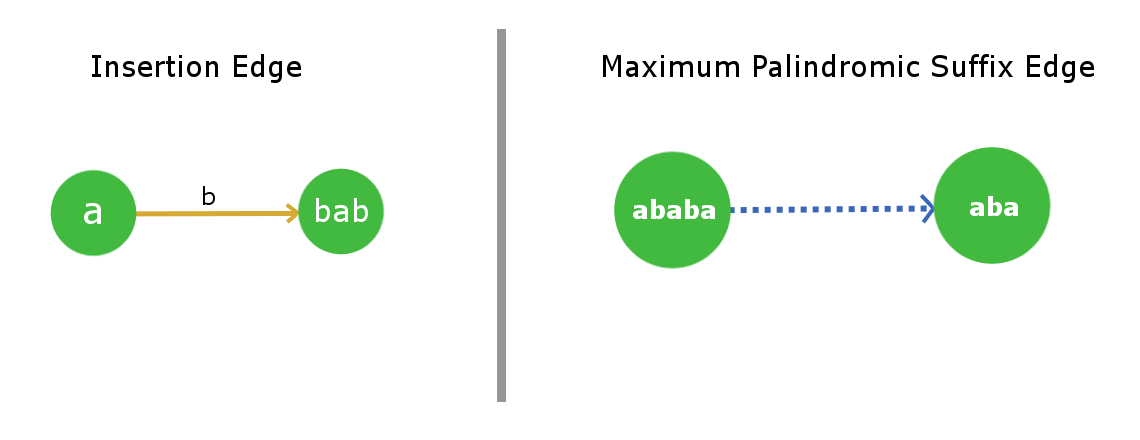Root Nodes and their convention: This tree/graph data structure will contain 2 root dummy nodes. More, precisely consider it as roots of two separate trees, which are linked together. Root-1 will be a dummy node which will describe a string for length = -1 ( you can easily infer from the implementation point of view that why we used so ). Root-2 will be a node which will describe a null string of length = 0. Root-1 has a suffix edge connected to itself(self-loop) as for any imaginary string of length -1 , its Maximum palindromic suffix will also be imaginary, so this is justified. Now Root-2 will also have its suffix edge connected to Root-1 as for a null string (length 0) there is no real palindromic suffix string of length less than 0.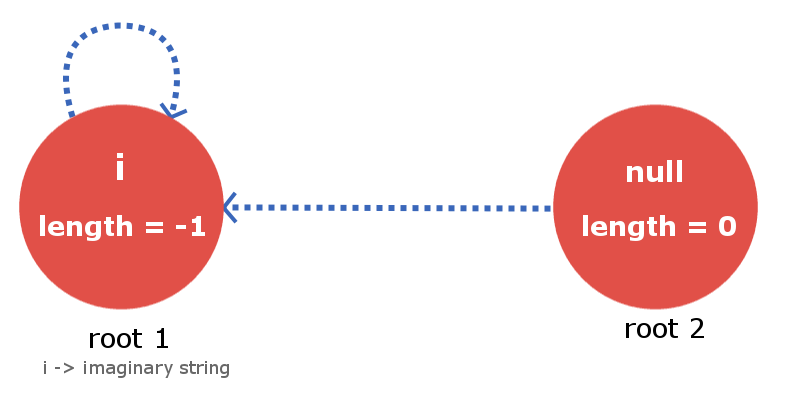Building the Palindromic Tree To build a Palindromic Tree, we will simply insert the characters in our string one by one till we reach its end and when we are done inserting we will be with our palindromic tree which will contain all the distinct palindromic substrings of the given strings. All we need to ensure is that, at every insertion of a new character, our palindromic tree maintains the above discussed feature. Let’s see how we can accomplish it. Let’s say we are given a string s with length l and we have inserted the string up till index k (k < l-1). Now, we need to insert the (k+1)th character. Insertion of (k+1)th character means insertion of a node that is longest palindrome ending at index (k+1). So, the longest palindromic string will be of form ( ‘s[k+1]’ + “X” + ‘s[k+1]’ ) and X will itself be a palindrome.Now the fact is that the string X lies at index < k+1 and is palindrome. So, it will already exist in our palindromic tree as we have maintained the very basic property of it saying that it will contain all the distinct palindromic substrings. So, to insert the character s[k+1] ,we only need to find the String X in our tree and direct the insertion edge from X with weight s[k+1] to a new node , which contains s[k+1]+X+s[k+1]. The main job now is to find the string X in efficient time. As we know that we are storing the suffix link for all the nodes. Therefore to track the node with string X we just need to move down the suffix link for the current node i.e the node which contains insertion of s[k]. See the below image for better understanding. The current node in the below figure tells that it is the largest palindrome that ends at index k after processing all the indices from 0 to k. The blue dotted path is the link of suffix edges from current node to other processed nodes in the tree. String X will exist in one of these nodes that lie on this chain of suffix link. All we need is to find it by iterating over it down the chain. To find the required node that contains the string X we will place the k+1 th character at the end of every node that lies in suffix link chain and check if first character of the corresponding suffix link string is equal to the k+1 th character. Once, we find the X string we will direct an insertion edge with weight s[k+1] and link it to the new node that contains largest palindrome ending at index k+1. The array elements between the brackets as described in the figure below are the nodes that are stored in the tree.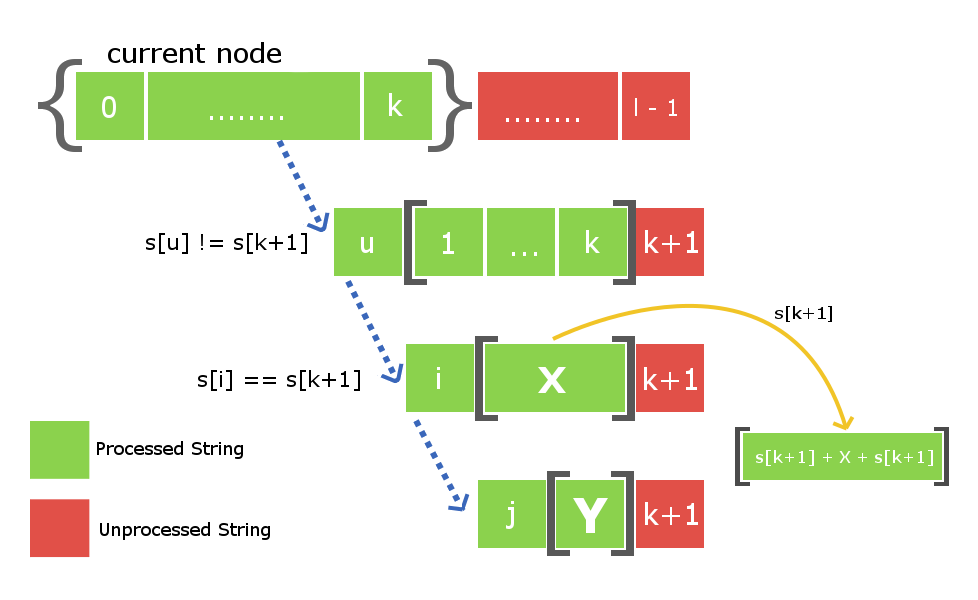There is one more thing left that is to be done. As we have created a new node at this s[k+1] insertion , therefore we will also have to connect it with its suffix link child. Once, again to do so we will use the above down the suffix link iteration from node X to find some new string Y such that s[k+1] + Y + s[k+1] is a largest palindromic suffix for the newly created node. Once, we find it we will then connect the suffix link of our newly created node with the node Y. Note : There are two possibilities when we find the string X. First possibility is that string s[k+1]Xs[k+1] do not exist in the tree and second possibility is if it already exists in the tree. In first case we will proceed the same way but in second case we will not create a new node separately but will just link the insertion edge from X to already existing S[k+1]+X+S[k+1] node in the tree. We also need not to add the suffix link because the node will already contain its suffix link. Consider a string s = “abba” with length = 4. At initial state we will have our two dummy root nodes one with length -1 ( some imaginary string i ) and second a null string with length 0. At this point we haven’t inserted any character in the tree. Root1 i.e root node with length -1 will be the current node from which insertion takes place.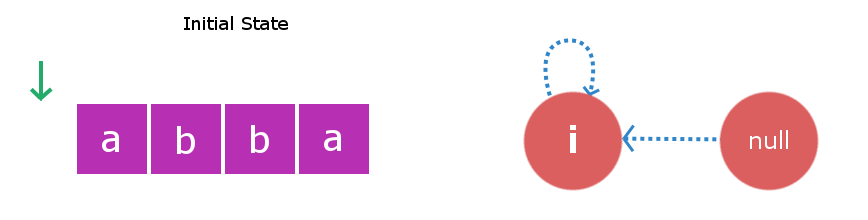Stage 1: We will insert s i.e ‘a‘.We will start checking from the current node i.e Root1. Inserting ‘a’ at start and end of a string with length -1 will yield to a string with length 1 and this string will be “a”. Therefore, we create a new node “a” and direct insertion edge from root1 to this new node. Now, largest palindromic suffix string for string of length 1 will be a null string so its suffix link will be directed to root2 i.e null string.Now the current node will be this new node “a”.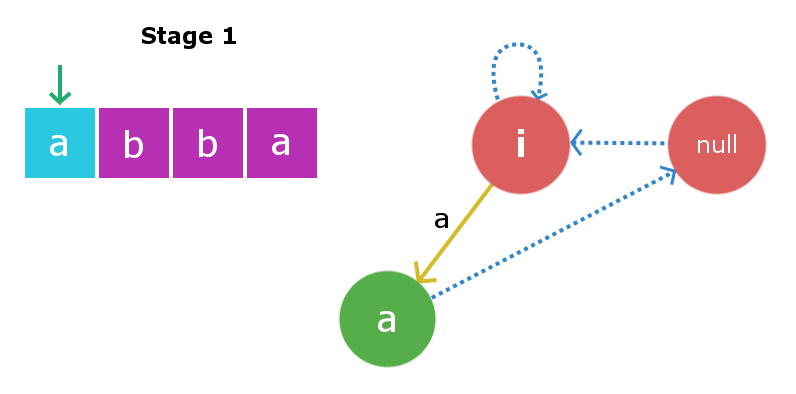Stage 2: We will insert s i.e ‘b‘. Insertion process will start from current node i.e “a” node. We will traverse the suffix link chain starting from current node till we find suitable X string , So here traversing the suffix link we again found root1 as X string. Once again inserting ‘b’ to string of length -1 will yield a string of length 1 i.e string “b”. Suffix link for this node will go to null string as described in above insertion. Now the current node will be this new node “b”.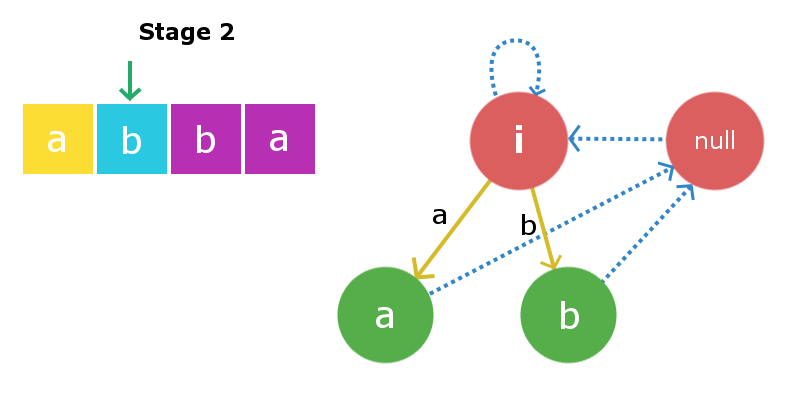Stage 3: We will insert s i.e ‘b‘. Once again starting from current node we will traverse its suffix link to find required X string. In this case it founds to be root2 i.e null string as adding ‘b’ at front and end of null string yields a palindrome “bb” of length 2. Therefore, we will create a new node “bb” and will direct the insertion edge from the null string to the newly created string. Now, the largest suffix palindrome for this current node will be node “b”. So, we will link the suffix edge from this newly created node to node “b”. Current node now becomes node “bb”.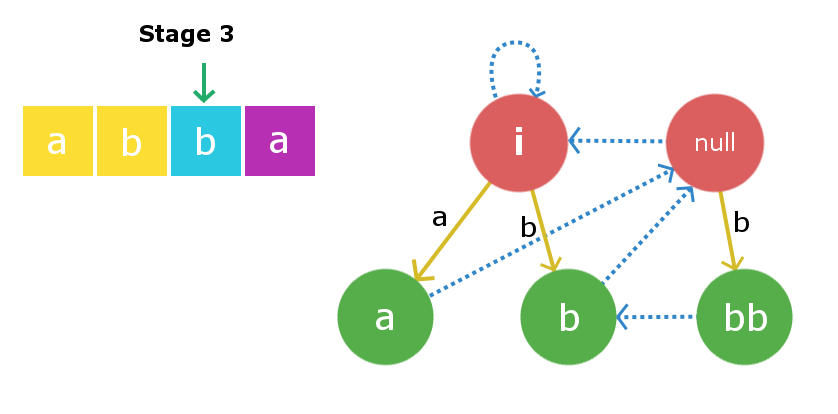Stage 4: We will insert s i.e ‘a‘. Insertion process begins with current node and in this case the current node itself is the largest X string such that s + X + s is palindrome. Therefore, we will create a new node “abba” and link the insertion edge from the current node “bb” to this newly created node with edge weight ‘a’. Now, the suffix the link from this newly created node will be linked to node “a” as that is the largest palindromic suffix.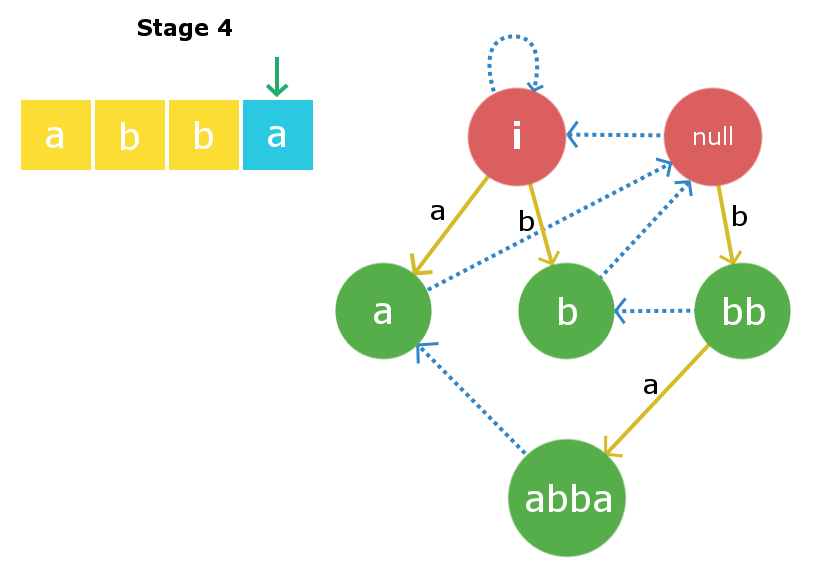The C++ implementation for the above implementation is given below :

## C++

 `// C++ program to demonstrate working of``// palindromic tree``#include "bits/stdc++.h"``using` `namespace` `std;` `#define MAXN  1000` `struct` `Node``{``    ``// store start and end indexes of current``    ``// Node inclusively``    ``int` `start, end;` `    ``// stores length of substring``    ``int` `length;` `    ``// stores insertion Node for all characters a-z``    ``int` `insertEdg;` `    ``// stores the Maximum Palindromic Suffix Node for``    ``// the current Node``    ``int` `suffixEdg;``};` `// two special dummy Nodes as explained above``Node root1, root2;` `// stores Node information for constant time access``Node tree[MAXN];` `// Keeps track the current Node while insertion``int` `currNode;``string s;``int` `ptr;` `void` `insert(``int` `idx)``{``//STEP 1//` `    ``/* search for Node X such that s[idx] X S[idx]``       ``is maximum palindrome ending at position idx``       ``iterate down the suffix link of currNode to``       ``find X */``    ``int` `tmp = currNode;``    ``while` `(``true``)``    ``{``        ``int` `curLength = tree[tmp].length;``        ``if` `(idx - curLength >= 1 and s[idx] == s[idx-curLength-1])``            ``break``;``        ``tmp = tree[tmp].suffixEdg;``    ``}` `    ``/* Now we have found X ....``     ``* X = string at Node tmp``     ``* Check : if s[idx] X s[idx] already exists or not*/``    ``if``(tree[tmp].insertEdg[s[idx]-``'a'``] != 0)``    ``{``        ``// s[idx] X s[idx] already exists in the tree``        ``currNode = tree[tmp].insertEdg[s[idx]-``'a'``];``        ``return``;``    ``}` `    ``// creating new Node``    ``ptr++;` `    ``// making new Node as child of X with``    ``// weight as s[idx]``    ``tree[tmp].insertEdg[s[idx]-``'a'``] = ptr;` `    ``// calculating length of new Node``    ``tree[ptr].length = tree[tmp].length + 2;` `    ``// updating end point for new Node``    ``tree[ptr].end = idx;` `    ``// updating the start for new Node``    ``tree[ptr].start = idx - tree[ptr].length + 1;`  `//STEP 2//` `    ``/* Setting the suffix edge for the newly created``       ``Node tree[ptr]. Finding some String Y such that``       ``s[idx] + Y + s[idx] is longest possible``       ``palindromic suffix for newly created Node.*/` `    ``tmp = tree[tmp].suffixEdg;` `    ``// making new Node as current Node``    ``currNode = ptr;``    ``if` `(tree[currNode].length == 1)``    ``{``        ``// if new palindrome's length is 1``        ``// making its suffix link to be null string``        ``tree[currNode].suffixEdg = 2;``        ``return``;``    ``}``    ``while` `(``true``)``    ``{``        ``int` `curLength = tree[tmp].length;``        ``if` `(idx-curLength >= 1 and s[idx] == s[idx-curLength-1])``            ``break``;``        ``tmp = tree[tmp].suffixEdg;``    ``}` `    ``// Now we have found string Y``    ``// linking current Nodes suffix link with s[idx]+Y+s[idx]``    ``tree[currNode].suffixEdg = tree[tmp].insertEdg[s[idx]-``'a'``];``}` `// driver program``int` `main()``{``    ``// initializing the tree``    ``root1.length = -1;``    ``root1.suffixEdg = 1;``    ``root2.length = 0;``    ``root2.suffixEdg = 1;` `    ``tree = root1;``    ``tree = root2;``    ``ptr = 2;``    ``currNode = 1;` `    ``// given string``    ``s = "abcbab";``    ``int` `l = s.length();` `    ``for` `(``int` `i=0; i

## Java

 `import` `java.util.*;` `class` `Node {``    ``int` `start, end;``    ``int` `length;``    ``int``[] insertEdg = ``new` `int``[``26``];``    ``int` `suffixEdg;``}` `public` `class` `PalindromicSubstrings {``    ``static` `final` `int` `MAXN = ``1000``;``    ``static` `Node root1 = ``new` `Node();``    ``static` `Node root2 = ``new` `Node();``    ``static` `Node[] tree = ``new` `Node[MAXN];``    ``static` `int` `currNode;``    ``static` `String s;``    ``static` `int` `ptr;` `    ``static` `void` `insert(``int` `idx) {``        ``int` `tmp = currNode;``        ``while` `(``true``) {``            ``int` `curLength = tree[tmp].length;``            ``if` `(idx - curLength >= ``1` `&& s.charAt(idx) == s.charAt(idx - curLength - ``1``))``                ``break``;``            ``tmp = tree[tmp].suffixEdg;``        ``}``        ``if` `(tree[tmp].insertEdg[s.charAt(idx) - ``'a'``] != ``0``) {``            ``currNode = tree[tmp].insertEdg[s.charAt(idx) - ``'a'``];``            ``return``;``        ``}``        ``ptr++;``        ``tree[tmp].insertEdg[s.charAt(idx) - ``'a'``] = ptr;``        ``tree[ptr] = ``new` `Node();``        ``tree[ptr].length = tree[tmp].length + ``2``;``        ``tree[ptr].end = idx;``        ``tree[ptr].start = idx - tree[ptr].length + ``1``;``        ``tmp = tree[tmp].suffixEdg;``        ``currNode = ptr;``        ``if` `(tree[currNode].length == ``1``) {``            ``tree[currNode].suffixEdg = ``2``;``            ``return``;``        ``}``        ``while` `(``true``) {``            ``int` `curLength = tree[tmp].length;``            ``if` `(idx - curLength >= ``1` `&& s.charAt(idx) == s.charAt(idx - curLength - ``1``))``                ``break``;``            ``tmp = tree[tmp].suffixEdg;``        ``}``        ``tree[currNode].suffixEdg = tree[tmp].insertEdg[s.charAt(idx) - ``'a'``];``    ``}` `    ``public` `static` `void` `main(String[] args) {``        ``root1.length = -``1``;``        ``root1.suffixEdg = ``1``;``        ``root2.length = ``0``;``        ``root2.suffixEdg = ``1``;``        ``tree[``1``] = root1;``        ``tree[``2``] = root2;``        ``ptr = ``2``;``        ``currNode = ``1``;``        ``s = ``"abcbab"``;``        ``int` `l = s.length();``        ``for` `(``int` `i = ``0``; i < l; i++)``            ``insert(i);``        ``System.out.println(``"All distinct palindromic substrings for "` `+ s + ``" :"``);``        ``for` `(``int` `i = ``3``; i <= ptr; i++) {``            ``System.out.print((i - ``2``) + ``") "``);``            ``for` `(``int` `j = tree[i].start; j <= tree[i].end; j++)``                ``System.out.print(s.charAt(j));``            ``System.out.println();``        ``}``    ``}``}`

## Python3

 `# python program to demonstrate working of``# palindromic tree``MAXN ``=` `1000`  `class` `Node:``    ``def` `__init__(``self``):``        ``# store start and end indexes of current``        ``# Node inclusively``        ``self``.start ``=` `0``        ``self``.end ``=` `0` `        ``# stores length of substring``        ``self``.length ``=` `0` `        ``# stores insertion Node for all characters a-z``        ``self``.insertEdg ``=` `[``0``]``*``26` `        ``# stores the Maximum Palindromic Suffix Node for``        ``# the current Node``        ``self``.suffixEdg ``=` `0`  `# two special dummy Nodes as explained above``root1 ``=` `Node()``root2 ``=` `Node()` `# stores Node information for constant time access``tree ``=` `[Node() ``for` `i ``in` `range``(MAXN)]` `# Keeps track the current Node while insertion``currNode ``=` `0``s ``=` `""``ptr ``=` `0`  `def` `insert(idx):``    ``global` `currNode, ptr` `    ``# STEP 1//` `    ``"""search for Node X such that s[idx] X S[idx]``    ``is maximum palindrome ending at position idx``    ``iterate down the suffix link of currNode to``    ``find X"""``    ``tmp ``=` `currNode``    ``while` `True``:``        ``curLength ``=` `tree[tmp].length``        ``if` `idx ``-` `curLength >``=` `1` `and` `s[idx] ``=``=` `s[idx``-``curLength``-``1``]:``            ``break``        ``tmp ``=` `tree[tmp].suffixEdg` `    ``"""Now we have found X ....``    ``X = string at Node tmp``    ``Check : if s[idx] X s[idx] already exists or not"""``    ``if` `tree[tmp].insertEdg[``ord``(s[idx])``-``ord``(``'a'``)] !``=` `0``:``        ``# s[idx] X s[idx] already exists in the tree``        ``currNode ``=` `tree[tmp].insertEdg[``ord``(s[idx])``-``ord``(``'a'``)]``        ``return` `    ``# creating new Node``    ``ptr ``+``=` `1` `    ``# making new Node as child of X with``    ``# weight as s[idx]``    ``tree[tmp].insertEdg[``ord``(s[idx])``-``ord``(``'a'``)] ``=` `ptr` `    ``# calculating length of new Node``    ``tree[ptr].length ``=` `tree[tmp].length ``+` `2` `    ``# updating end point for new Node``    ``tree[ptr].end ``=` `idx` `    ``# updating the start for new Node``    ``tree[ptr].start ``=` `idx ``-` `tree[ptr].length ``+` `1` `    ``# STEP 2//` `    ``"""Setting the suffix edge for the newly created``    ``Node tree[ptr]. Finding some String Y such that``    ``s[idx] + Y + s[idx] is longest possible``    ``palindromic suffix for newly created Node."""` `    ``tmp ``=` `tree[tmp].suffixEdg` `    ``# making new Node as current Node``    ``currNode ``=` `ptr``    ``if` `tree[currNode].length ``=``=` `1``:``        ``# if new palindrome's length is 1``        ``# making its suffix link to be null string``        ``tree[currNode].suffixEdg ``=` `2``        ``return``    ``while` `True``:``        ``curLength ``=` `tree[tmp].length``        ``if` `idx``-``curLength >``=` `1` `and` `s[idx] ``=``=` `s[idx``-``curLength``-``1``]:``            ``break``        ``tmp ``=` `tree[tmp].suffixEdg` `    ``# Now we have found string Y``    ``# linking current Nodes suffix link with s[idx]+Y+s[idx]``    ``tree[currNode].suffixEdg ``=` `tree[tmp].insertEdg[``ord``(s[idx])``-``ord``(``'a'``)]`  `# driver program``if` `__name__ ``=``=` `'__main__'``:``    ``# initializing the tree``    ``root1.length ``=` `-``1``    ``root1.suffixEdg ``=` `1``    ``root2.length ``=` `0``    ``root2.suffixEdg ``=` `1` `    ``tree[``1``] ``=` `root1``    ``tree[``2``] ``=` `root2``    ``ptr ``=` `2``    ``currNode ``=` `1` `    ``# given string``    ``s ``=` `"abcbab"``    ``l ``=` `len``(s)` `    ``for` `i ``in` `range``(l):``        ``insert(i)` `    ``# printing all of its distinct palindromic``    ``# substring``    ``print``(``"All distinct palindromic substring for "``, s, ``" : "``)``    ``for` `i ``in` `range``(``3``, ptr``+``1``):``        ``print``(i``-``2``, ``") "``, end``=``"")``        ``for` `j ``in` `range``(tree[i].start, tree[i].end``+``1``):``            ``print``(s[j], end``=``"")``        ``print``()`

## C#

 `// C# program to find all distinct palindromic substrings of a string` `using` `System;` `public` `class` `Program``{``    ``// Define the maximum size of the tree``    ``private` `const` `int` `MAXN = 1000;` `    ``// Define the node class for the tree``    ``private` `class` `Node``    ``{``        ``public` `int` `start = 0;``        ``public` `int` `end = 0;``        ``public` `int` `length = 0;``        ``public` `int``[] insertEdg = ``new` `int``;``        ``public` `int` `suffixEdg = 0;``    ``}` `    ``// Define the root nodes and other variables``    ``private` `static` `Node root1 = ``new` `Node();``    ``private` `static` `Node root2 = ``new` `Node();``    ``private` `static` `Node[] tree = ``new` `Node[MAXN];``    ``private` `static` `int` `currNode = 0;``    ``private` `static` `string` `s = ``""``;``    ``private` `static` `int` `ptr = 0;` `    ``// Function to insert a new palindrome into the tree``    ``private` `static` `void` `Insert(``int` `idx)``    ``{``        ``int` `tmp = currNode;` `        ``// Traverse the tree to find the proper place to insert the new palindrome``        ``while` `(``true``)``        ``{``            ``int` `curLength = tree[tmp].length;` `            ``if` `(idx - curLength >= 1 && s[idx] == s[idx - curLength - 1])``            ``{``                ``break``;``            ``}` `            ``tmp = tree[tmp].suffixEdg;``        ``}` `        ``// If the new palindrome already exists in the tree, return``        ``if` `(tree[tmp].insertEdg[s[idx] - ``'a'``] != 0)``        ``{``            ``currNode = tree[tmp].insertEdg[s[idx] - ``'a'``];``            ``return``;``        ``}` `        ``// Create a new node for the new palindrome``        ``ptr += 1;``        ``if` `(ptr < MAXN && tree[ptr] == ``null``)``        ``{``            ``tree[ptr] = ``new` `Node();``        ``}``        ``tree[tmp].insertEdg[s[idx] - ``'a'``] = ptr;``        ``tree[ptr].length = tree[tmp].length + 2;``        ``tree[ptr].end = idx;``        ``tree[ptr].start = idx - tree[ptr].length + 1;` `        ``// Update the current node and suffix edge of the new palindrome``        ``tmp = tree[tmp].suffixEdg;``        ``currNode = ptr;` `        ``if` `(tree[currNode].length == 1)``        ``{``            ``tree[currNode].suffixEdg = 2;``            ``return``;``        ``}` `        ``// Traverse the tree again to update the suffix edge of the new palindrome``        ``while` `(``true``)``        ``{``            ``int` `curLength = tree[tmp].length;` `            ``if` `(idx - curLength >= 1 && s[idx] == s[idx - curLength - 1])``            ``{``                ``break``;``            ``}` `            ``tmp = tree[tmp].suffixEdg;``        ``}` `        ``tree[currNode].suffixEdg = tree[tmp].insertEdg[s[idx] - ``'a'``];``    ``}` `    ``public` `static` `void` `Main()``    ``{``        ``// Initialize the root nodes and other variables``        ``root1.length = -1;``        ``root1.suffixEdg = 1;``        ``root2.length = 0;``        ``root2.suffixEdg = 1;``        ``tree = root1;``        ``tree = root2;``        ``ptr = 2;``        ``currNode = 1;``        ``s = ``"abcbab"``;``        ``int` `l = s.Length;` `        ``// Insert each possible palindrome into the tree``        ``for` `(``int` `i = 0; i < l; i++)``        ``{``            ``Insert(i);``        ``}``        ``Console.Write(``"All distinct palindromic substring for "` `+ s + ``" : \n"``);` `        ``for` `(``int` `i = 3; i <= ptr; i++)``        ``{``            ``Console.Write(i - 2 + ``") "``);` `            ``for` `(``int` `j = tree[i].start; j <= tree[i].end; j++)``            ``{``                ``Console.Write(s[j]);``            ``}` `            ``Console.Write(``"\n"``);``        ``}``    ``}``}` `// This code is contributed by Amit Mangal.`

## Javascript

 `const MAXN = 1000;` `class Node {``    ``constructor() {``        ``// store start and end indexes of current``        ``// Node inclusively``        ``this``.start = 0;``        ``this``.end = 0;` `        ``// stores length of substring``        ``this``.length = 0;` `        ``// stores insertion Node for all characters a-z``        ``this``.insertEdg = ``new` `Array(26).fill(0);` `        ``// stores the Maximum Palindromic Suffix Node for``        ``// the current Node``        ``this``.suffixEdg = 0;``    ``}``}` `// two special dummy Nodes as explained above``const root1 = ``new` `Node();``const root2 = ``new` `Node();` `// stores Node information for constant time access``const tree = ``new` `Array(MAXN);` `// Keeps track the current Node while insertion``let currNode = 0;``let s = ``''``;``let ptr = 0;` `function` `insert(idx) {``    ``// STEP 1``    ``/* search for Node X such that s[idx] X S[idx]``       ``is maximum palindrome ending at position idx``       ``iterate down the suffix link of currNode to``       ``find X */``    ``let tmp = currNode;``    ``while` `(``true``) {``        ``const curLength = tree[tmp].length;``        ``if` `(idx - curLength >= 1 && s[idx] === s[idx - curLength - 1])``            ``break``;``        ``tmp = tree[tmp].suffixEdg;``    ``}` `    ``/* Now we have found X ....``     ``* X = string at Node tmp``     ``* Check : if s[idx] X s[idx] already exists or not*/``    ``if` `(tree[tmp].insertEdg[s.charCodeAt(idx) - ``'a'``.charCodeAt(0)] !== 0) {``        ``// s[idx] X s[idx] already exists in the tree``        ``currNode = tree[tmp].insertEdg[s.charCodeAt(idx) - ``'a'``.charCodeAt(0)];``        ``return``;``    ``}` `    ``// creating new Node``    ``ptr++;` `    ``// making new Node as child of X with``    ``// weight as s[idx]``    ``tree[tmp].insertEdg[s.charCodeAt(idx) - ``'a'``.charCodeAt(0)] = ptr;` `    ``// calculating length of new Node``    ``tree[ptr] = ``new` `Node();``    ``tree[ptr].length = tree[tmp].length + 2;` `    ``// updating end point for new Node``    ``tree[ptr].end = idx;` `    ``// updating the start for new Node``    ``tree[ptr].start = idx - tree[ptr].length + 1;` `    ``// STEP 2``    ``/* Setting the suffix edge for the newly created``       ``Node tree[ptr]. Finding some String Y such that``       ``s[idx] + Y + s[idx] is longest possible``       ``palindromic suffix for newly created Node.*/``    ``tmp = tree[tmp].suffixEdg;` `    ``// making new Node as current Node``    ``currNode = ptr;``    ``if` `(tree[currNode].length === 1) {``        ``// if new palindrome's length is 1``        ``// making its suffix link to be null string``        ``tree[currNode].suffixEdg = 2;``        ``return``;``    ``}``    ``while` `(``true``) {``        ``const curLength = tree[tmp].length;``        ``if` `(idx - curLength >= 1 && s[idx] === s[idx - curLength - 1])``            ``break``;``        ``tmp = tree[tmp].suffixEdg;``    ``}` `    ``// Now we have found string Y``    ``// linking current Nodes suffix link with s[idx]+Y+s[idx]``    ``tree[currNode].suffixEdg = tree[tmp].insertEd`

Output

```All distinct palindromic substrings for abcbab :
1) a
2) b
3) c
4) bcb
5) abcba
6) bab```

Time Complexity The time complexity for the building process will be O(k*n), here “n” is the length of the string and ‘k‘ is the extra iterations required to find the string X and string Y in the suffix links every time we insert a character.Let’s try to approximate the constant ‘k’. We shall consider a worst case like s = “aaaaaabcccccccdeeeeeeeeef”. In this case for similar streak of continuous characters it will take extra 2 iterations per index to find both string X and Y in the suffix links , but as soon as it reaches some index i such that s[i]!=s[i-1] the left most pointer for the maximum length suffix will reach its rightmost limit. Therefore, for all i when s[i]!=s[i-1] , it will cost in total n iterations(summing over each iteration) and for rest i when s[i]==s[i-1] it takes 2 iteration which sums up over all such i and takes 2*n iterations. Hence, approximately our complexity in this case will be O(3*n) ~ O(n).So, we can roughly say that the constant factor ‘k’ will be very less. Therefore, we can consider the overall complexity to be linear O(length of string). You may refer the reference links for better understanding. References :